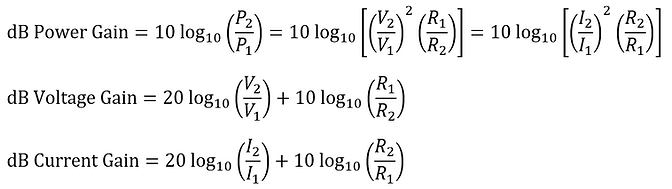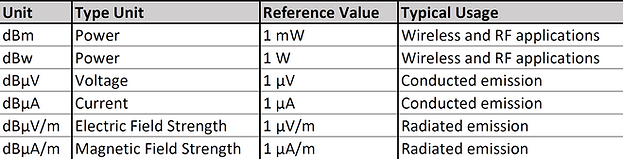top of page

## 1  The Decibel

The decibel is defined as a ratio of two quantities, typically power, voltage, or current. In the field of EMC, the decibel must be understood. Here are the most important points:

Gain [dB] and Loss [dB].

Let's have a look at the amplifier or damping network below.The power, voltage, and current gain of this network can be expressed in [dB] as [1.1]:If R1 and R2 are equal (typically 50Ω), then the following term is 0:And we can write the following for power/voltage/current gain:Points to remember when it comes to the calculation of gain and loss in decibel:

• Amplification. If P2 is bigger than P1, the gain value in [dB] is positive. This means if there is amplification, the power gain in [dB] is positive.

• Damping. If P2 is smaller than P1, the gain value in [dB] is negative. This means if there is a power loss, the power gain in [dB] is negative.

• Cut-off Frequency. At the cut-off frequency, the output power (P2) is half the input power (P1). And the power/voltage/current gains are all -3dB.• Ratio to [dB]. If power increases by factor 2, the power/voltage/current gains increase by +3dB. If power increases by factor 10, the power/voltage/current gains increase by +10dB. To get an overview, have a look at our power/voltage/current ratios table below.

Absolute Levels [dBm, dBµV, dBµA].

The most common absolute power, voltage and current levels in EMC are [dBm], [dBµV] and [dBµA]. They are calculated like this [1.1]:The most common absolute power, voltage and current levels in EMC are [dBm], [dBµV] and [dBµA]. They are calculated like this:For example, a negative dBm-value means that the power level is <1mW. 0dBm = 1mW and a dBm-value bigger than 0 means that the power is higher than 1mW. The same for dBµV and dBµA: 0dBµV = 1µV (0dBµA = 1µA), a negative dBµV-value means <1µV, and a positive dBµV-value means >1µV.Conversion formulas and tables.

Find below tables and formulas to convert between the different dB-units and from linear ratio values to dB-values and vice versa. In addition, you can download an Excel sheet, which contains conversion calculations and more [1.2].Gain and Loss
Absolute Levels
Unit Conversion
bottom of page